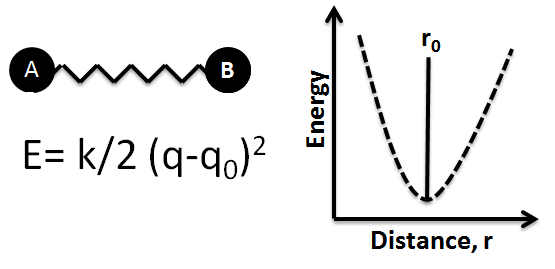Bond Lengths

Learning Objective

• Compare bond lengths using knowledge of periodic trends

Key Points

• Bond length is the experimentally determined average distance between two bonded atoms.
• Bonded atoms vibrate due to thermal energy available in the surroundings.
• Bond lengths are typically in the range of 100-200 pm (1-2 Å).
• As a general trend, bond length decreases across a row in the periodic table and increases down a group.
• Atoms with multiple bonds between them have shorter bond lengths than singly bonded ones.

Terms

• bond lengthIn molecular geometry, bond length or bond distance is the average distance between nuclei of two bonded atoms in a molecule.
• covalent radiusThe radius of an atom when covalently bonded to other atoms.

The Distance Between Two Bonded Atoms

The distance between two atoms participating in a bond, known as the bond length, can be determined experimentally. X-ray diffraction of molecular crystals allows for the determination of the three-dimensional structure of molecules and the precise measurement of internuclear distances. Various spectroscopic methods also exist for estimating the bond length between two atoms in a molecule.

Bonds Are Not Static Structures

The bond length is the average distance between the nuclei of two bonded atoms in a molecule. This is because a chemical bond is not a static structure, but the two atoms actually vibrate due to thermal energy available in the surroundings at any non-zero Kelvin temperature. A bond can be modeled as two balls connected by a spring: stretching or compressing the spring initiates a back-and-forth motion with respect to the equilibrium positions of the balls. Measured bond lengths are the distance between those unperturbed, or equilibrium, positions of the balls, or atoms.Ball-and-spring model of a chemical bondA bond between two atoms can be thought of as a spring with two balls attached to it. Any stretch or compression of the spring will initiate oscillations of the atoms with respect to their equilibrium (unperturbed) positions. The potential energy function for this system is also indicated. The minimum energy occurs at the equilibrium distance r0, which is where the bond length is measured.

Bond Length Values

Bond lengths have traditionally been expressed in Ångstrom units, but picometers are sometimes preferred (1 Å = 10-10 m = 100 pm). Bonds lengths are typically in the range of 1-2 Å, or 100-200 pm. Even though the bond vibrates, equilibrium bond lengths can be determined experimentally to within ±1 pm. The actual distance between two atoms in a molecule depends on factors such as the orbital hybridization and the electronic nature of its components.

Bonds involving hydrogen can be quite short; the shortest bond of all, H–H, is only 74 pm. The covalent radius of an atom is determined by halving the bond distance between two identical atoms. Based on data for the H2 molecule, the covalent radius of H is 37 pm. Covalent radii can be used to estimate the bond distance between two different atoms; it is the sum of the individual covalent radii.

Periodic Trends

Generally, when we consider a bond between a given atom and a varying atomic bonding partner, the bond length decreases across a period in the periodic table, and increases down a group. This trend is identical to that of the atomic radius.

Atoms with multiple bonds between them have shorter bond lengths than singly bonded ones; this is a major criterion for experimentally determining the multiplicity of a bond. For example, the bond length of $C - C$ is 154 pm; the bond length of $C = C$ is 133 pm; and finally, the bond length of $C \equiv C$ is 120 pm.